#【数据分析】不容错过的Pandas小技巧

0
0
01. 云栖社区>
2. 博客>
3. 正文

## 【数据分析】不容错过的Pandas小技巧

Pandas实用技巧

Pandas 在这一点上其实十分友好，只需添加一行代码。

DataFrame 转 HTML

import numpy as np
import pandas as pd
import random

n = 10
df = pd.DataFrame(

{
"col1": np.random.random_sample(n),
"col2": np.random.random_sample(n),
"col3": [[random.randint(0, 10) for _ in range(random.randint(3, 5))] for _ in range(n)],
}

)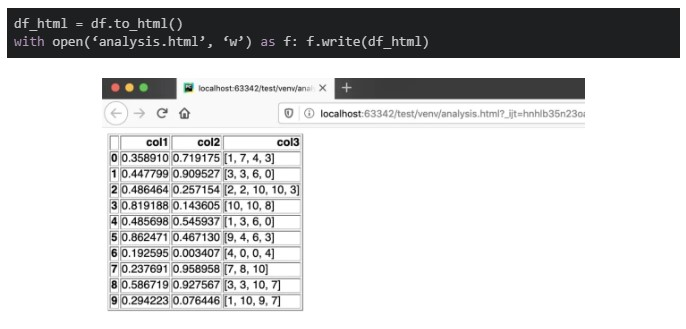DataFrame 转 LaTeX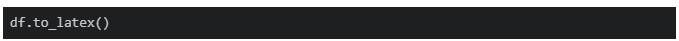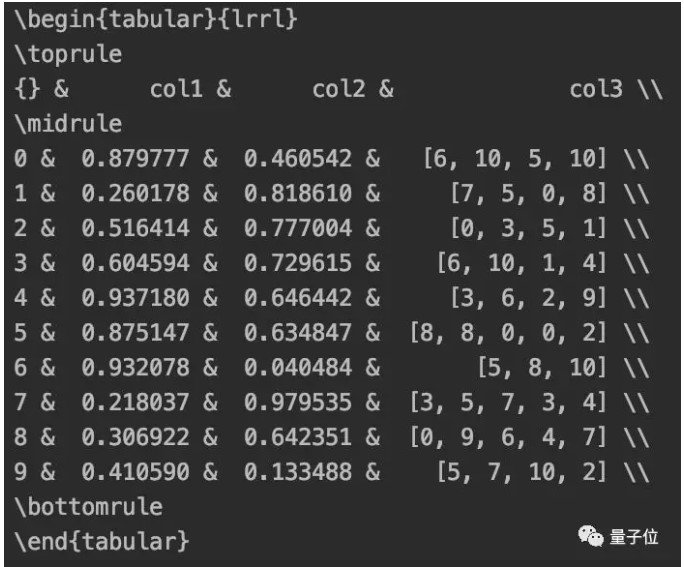DataFrame 转 Markdown

Pandas 同样为你考虑到了这一点：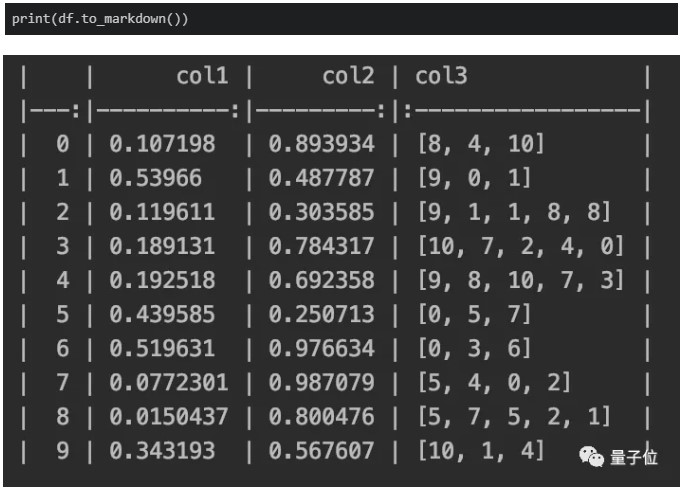DataFrame 转 Excel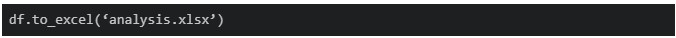DataFrame 转字符串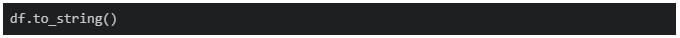5个鲜为人知的Pandas技巧

1、data_range

Pandas 的 data_range 覆盖了这一需求。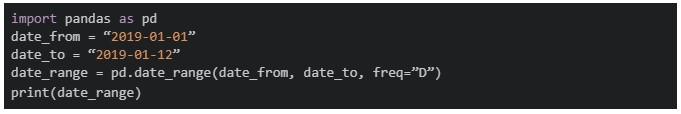freq = “D”/“M”/“Y”，该函数就会分别返回按天、月、年递增的日期。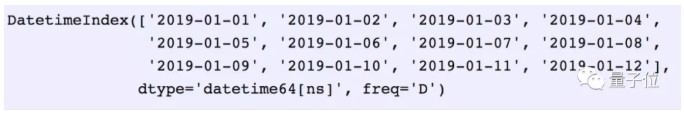2、合并数据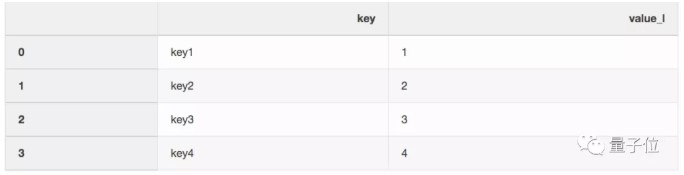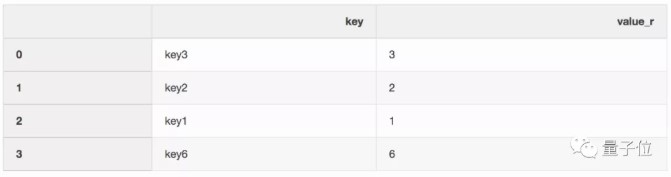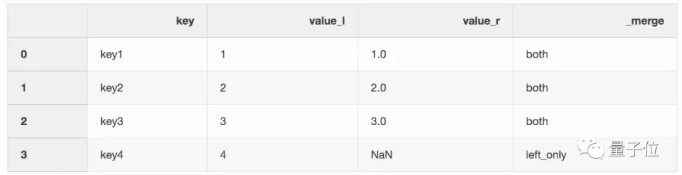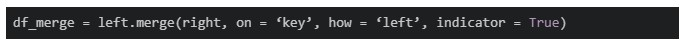3、最近合并（Nearest merge）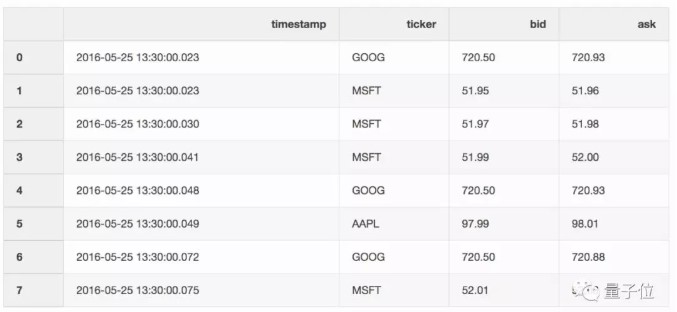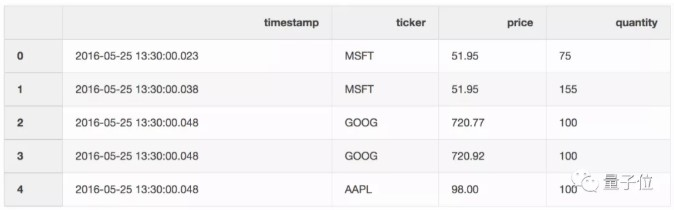pd.merge_asof(trades, quotes, on=”timestamp”, by=’ticker’, tolerance=pd.Timedelta(‘10ms’), direction=‘backward’)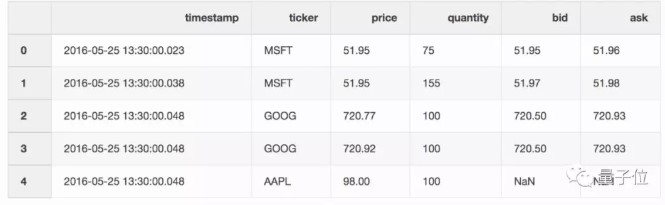4、创建Excel报告

import numpy as np
import pandas as pd

df = pd.DataFrame(np.array([[1, 2, 3], [4, 5, 6], [7, 8, 9]]), columns=["a", "b", "c"])

report_name = 'example_report.xlsx'
sheet_name = 'Sheet1'
writer = pd.ExcelWriter(report_name, engine='xlsxwriter')
df.to_excel(writer, sheet_name=sheet_name, index=False)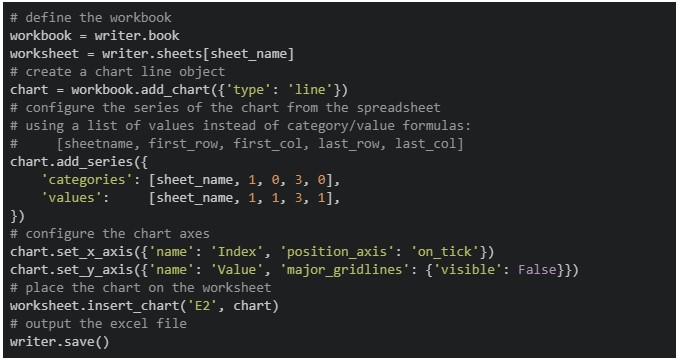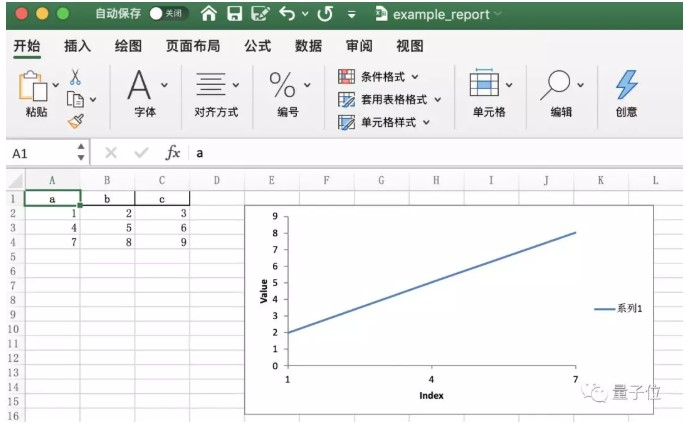5、节省磁盘空间

Pandas在保存数据集时，可以对其进行压缩，其后以压缩格式进行读取。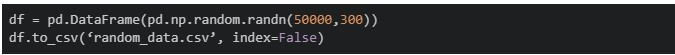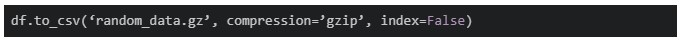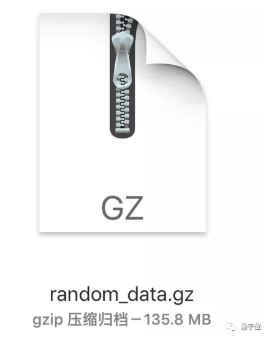gzip压缩文件可以直接读取：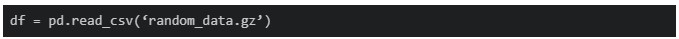Talk is cheap, show me the code。学会了，就用起来吧

【云栖号在线课堂】每天都有产品技术专家分享！

【云栖号在线课堂 社群】https://c.tb.cn/F3.Z8gvnK

+ 关注